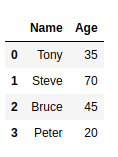# How to Convert Pandas DataFrame into a List?

Let’s discuss how to convert Pandas dataframe to List. First, let’s create a Basic DataFrame:

## Python3

 `import` `pandas as pd   ` ` `  `# Creating a dictionary to store data ` `data ``=` `{``'Name'``:[``'Tony'``, ``'Steve'``, ``'Bruce'``, ``'Peter'` `], ` `        ``'Age'``: [``35``, ``70``, ``45``, ``20``] }   ` ` `  `# Creating DataFrame   ` `df ``=` `pd.DataFrame(data)   ` ` `  `# Print the dataframe  ` `df`

Output :At times, you may need to convert your pandas dataframe to List. To accomplish this task, ‘ tolist() ‘ function can be used. Below is a basic example to use this function and convert the required DataFrame into a List.

## Python3

 `df.values.tolist()`

Output :

`[['Tony', 35], ['Steve', 70], ['Bruce', 45], ['Peter', 20]]`

Here, Each inner list contains all the columns of a particular row.

Pandas DataFrame can be converted into lists in multiple ways. Let’s have a look at different ways of converting a DataFrame one by one.

Method #1: Converting a DataFrame to List containing all the rows of a particular column:

## Python3

 `import` `pandas as pd   ` ` `  `# Creating a dictionary to store data ` `data ``=` `{``'Name'``:[``'Tony'``, ``'Steve'``, ``'Bruce'``, ``'Peter'` `] , ` `        ``'Age'``: [``35``, ``70``, ``45``, ``20``] }   ` ` `  `# Creating DataFrame   ` `df ``=` `pd.DataFrame(data)   ` ` `  `# Convering DataFrame to a list containg ` `# all the rows of column 'Name' ` `names ``=` `df[``'Name'``].tolist() ` ` `  `# Printing the converted list. ` `print``(names)`

Output:

`['Tony', 'Steve', 'Bruce', 'Peter']`

Method #2: Converting a DataFrame to Nested List containing all the rows of all the columns:

## Python3

 `import` `pandas as pd   ` ` `  `# Creating a dictionary to store data ` `data ``=` `{``'Name'``:[``'Tony'``, ``'Steve'``, ``'Bruce'``, ``'Peter'` `] , ` `        ``'Age'``: [``35``, ``70``, ``45``, ``20``] }   ` ` `  `# Creating DataFrame ` `df ``=` `pd.DataFrame(data)  ` ` `  `# Creating an empty list ` `res``=``[] ` ` `  `# Iterating through the columns of  ` `# dataframe ` `for` `column ``in` `df.columns: ` `     `  `    ``# Storing the rows of a column  ` `    ``# into a temporary list ` `    ``li ``=` `df[column].tolist() ` `     `  `    ``# appending the temporary list ` `    ``res.append(li) ` `     `  `# Printing the final list ` `print``(res)`

Output:

`[['Tony', 'Steve', 'Bruce', 'Peter'], [35, 70, 45, 20]]`

Method #3: Converting a DataFrame to a list that contains lists having all the columns of a row.

## Python3

 `import` `pandas as pd   ` ` `  `# Creating a dictionary to store data ` `data ``=` `{``'Name'``:[``'Tony'``, ``'Steve'``, ``'Bruce'``, ``'Peter'` `] , ` `        ``'Age'``: [``35``, ``70``, ``45``, ``20``] }   ` ` `  `# Creating DataFrame ` `df ``=` `pd.DataFrame(data)   ` ` `  `# Converting dataframe to list ` `li ``=` `df.values.tolist() ` ` `  `# Printing list ` `print``(li)`

Output :

`[['Tony', 35], ['Steve', 70], ['Bruce', 45], ['Peter', 20]]`

Method #4: Converting a DataFrame to a list that contains lists having all the columns of a row along with column names.

## Python3

 `import` `pandas as pd   ` ` `  `# Creating a dictionary to store data ` `data ``=` `{``'Name'``:[``'Tony'``, ``'Steve'``, ``'Bruce'``, ``'Peter'` `] ,  ` `        ``'Age'``: [``35``, ``70``, ``45``, ``20``] }   ` ` `  `# Creating DataFrame ` `df ``=` `pd.DataFrame(data)   ` ` `  `# Converting dataframe to list ` `li ``=` `[df.columns.values.tolist()] ``+` `df.values.tolist() ` ` `  `# Printing list ` `print``(li)`

Output:

[[‘Name’, ‘Age’], [‘Tony’, 35], [‘Steve’, 70], [‘Bruce’, 45], [‘Peter’, 20]]

My Personal Notes arrow_drop_upCheck out this Author's contributed articles.

If you like GeeksforGeeks and would like to contribute, you can also write an article using contribute.geeksforgeeks.org or mail your article to contribute@geeksforgeeks.org. See your article appearing on the GeeksforGeeks main page and help other Geeks.

Please Improve this article if you find anything incorrect by clicking on the "Improve Article" button below.

Article Tags :

Be the First to upvote.

Please write to us at contribute@geeksforgeeks.org to report any issue with the above content.